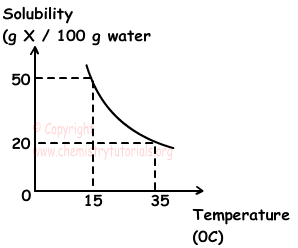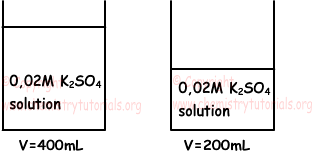## Solutions Exam3 and Problem Solutions

Solutions Exam3 and  Problem Solutions

1. If we add 40 g sugar to 200 g % 20 sugar water  solution, what would be the new concentration of solution?

Solution:

Amount of sugar in first solution is;

100 g water includes 20 g sugar

200 g water includes ? g sugar

¯¯¯¯¯¯¯¯¯¯¯¯¯¯¯¯¯¯¯¯¯¯¯¯¯¯

?=40 g sugar

New concentration of solution can be found;

(200+40) g solution includes 80(40 + 40) g sugar

100 g solution includes how much sugar?

¯¯¯¯¯¯¯¯¯¯¯¯¯¯¯¯¯¯¯¯¯¯¯¯¯¯¯¯¯¯¯¯¯¯¯¯¯¯

?=33,3

Thus new solution has concentration :% 33,3

2. 1 L and 2M NaBr is mixed with 4 L and 0,5 M NaBr. Find final concentration of this mixture?

Solution:

M1=2M, M2=0,5M

V1=1L, V2=4L and Vfinal=1L + 4L=5L

Mfinal=?

We use following formula to find concentration of mixtures;

M1.V1 + M2.V2 = Mfinal.Vfinal

2.1 + 0,5.4=Mfinal.5

Mfinal=0,8 M

3. Solubility of X solid in water vs. temperature graph is given below. When we heat 300 g saturated solution at 15 0C to 35 0C, 0,6 mol X is crystallized, find molar mass of X.Solution:

As you can see from the graph, at 15 0C, 100 g water can dissolve 50 g X. Thus, at this temperature there are 100 g water in 150 g saturated solution.

At 15 0C;

150 g saturated solution has 100 g water

300 g saturated solution has ? g water

¯¯¯¯¯¯¯¯¯¯¯¯¯¯¯¯¯¯¯¯¯¯¯¯¯¯¯¯¯¯¯¯¯¯¯¯¯¯

?=200 g water

According to graph, 100 g water can dissolve 50 g X at 15 0C and 20 g X at 35 0C. Then, when solution prepared by using 100 g water at 15 0C is heated to 35 0C;

50 - 20 = 30 g X is crystallized.

15 0C → 35 0C;

In 100 g water 30 g X is crystallized

In 200 g water ? g X is crystallized

¯¯¯¯¯¯¯¯¯¯¯¯¯¯¯¯¯¯¯¯¯¯¯¯¯¯¯¯¯¯¯¯

?=60 g X is crystallized.

Mole of crystallized X is 0,6 so;

0,6 mol X is 60 g

1 mol X is ? g

¯¯¯¯¯¯¯¯¯¯¯¯¯¯¯

?= 100 g (molar mass of X)

4. Which one of the following properties are same for given two solutions;

I. Concentration percent by mass

II. Mole of dissolved K2SO4

III. DensitySolution:

I. There are K2SO4 solutions in both containers. Since their molar concentrations are equal their concentrations percent by mass are also equal. I is true.

II. Mole of K2SO4 in first container;

nK2SO4=V.M=(0,4).(0,02)=0,008mol

Mole of K2SO4 in second container;

nK2SO4=V.M=(0,2).(0,02)=0,004mol

Thus, mole of dissolved K2SO4 are different. II is false.

III. Density of solutions depends on concentration of it. Since their concentrations are equal, the have also equal densities. III is true.

5. :Which one of the following statements is false for solutions?

I. They are homogeneous mixtures.

II. Amount of solute in unit volume of solution is called "concentration".

III. Solubility of solids in liquids increases with temperature in general.

IV. They includes at least to matters that one of them must be liquid.

Solution:

I, II and III are true for solutions. However, solutions are homogeneous mixtures of at least two matters, they can be solids, liquids or gases. IV is false, there is no such condition.

Related